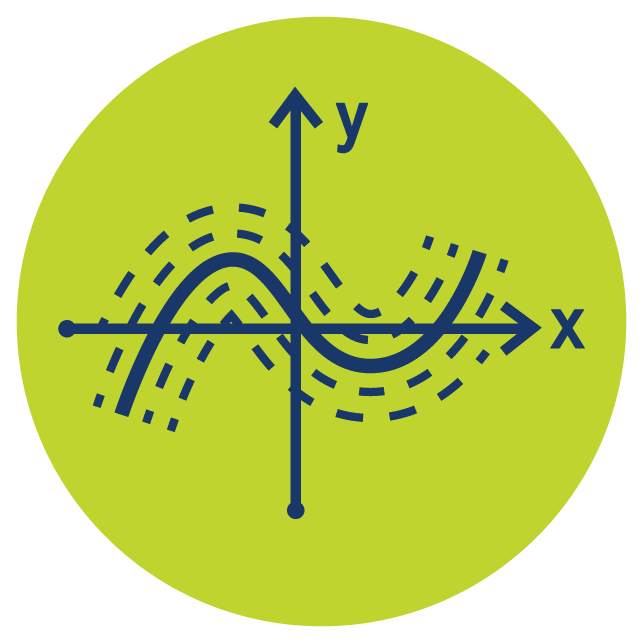Select Page# Differential Equations

The Differential Equations online course helps you master a range of types of differential equations. Differential equations are the equations of change and crucial in solving many real world problems in science, technology, engineering and others. Students pursuing these areas should develop strong differential equation solving skill. View our classroom with this PREVIEW or learn more and start your FREE Differential Equations one week TRIAL.

### Who is the Differential Equations Online Course For?

Differential Equations can be taken as a stand-alone course but may appeal to students following careers in Math, engineering or science. You should have some experience of differential and integral Calculus before taking Differential Equations. View the CLASSROOM.

### What is Taught in the Differential Equations Online Course?

Euler’s Method, Seperable Differential Equations, 1st & 2nd Order Differential Equations, Reducible Differential Equations, Exact Differential Equations, Laplace Transforms, Series Solutions. Differential Equations is especially useful if you are new to this material. However, it is equally helpful for students looking to refresh their knowledge and practice. View the full SYLLABUS.

### What Will I Learn in This Course?

The Differential Equations online course firstly teaches students basic principles of differential equations. Subsequently students learn standard and advanced techniques, and numerical methods. These techniques are used to solve a wide range of types of differential equation.

### How Does Differential Equations Compare to Calculus?

Differential equations mostly use Calculus techniques so students should have experience of these before studying differential equations. This background is certainly key to success in understanding and solving differential equations. Learn more about CALCULUS 1 and CALCULUS 2.

### What’s Next?

Differential Equations are used in many disciplines, particularly where real-world scenarios are modelled using Differential Equations. For that reason the skills developed in this course are of particular interest to students following careers in science, technology or engineering.

## Core Calculus & Beyond Course Bundle

Access all 6 courses in the bundle Core Calculus & Beyond to easily review older topics or jump ahead when ready.